The Vietnamese Mathematical Hobby Group

Math Test for Year 9/10 Students # 1

1. Factorise the following:

(a)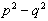(b)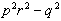(c)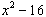(d)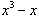2. Factorise each of the following:

(a)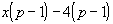(b)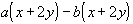(c)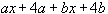3. Simplify the following:

(a)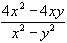(b)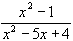(c)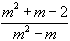4. Express each of the following as a single fraction:

(a)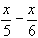(b)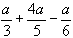(c)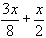5. Solve the following inequalities:

(a)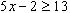(b)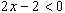(c)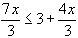6. Simplify the following surds
(a)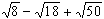(b)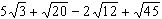(c)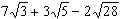7. Express each of the following in simplest surd form with rational denominator

(a)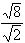(b)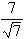(c)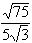8. If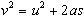, find

(a) s when u = 5, a = 6 and t = 2.4

(b) a when s = 50, t = 2.5

9. Express the following in the form y = mx + c, and hence state the gradient of each

(a) 2x + 3y = 4 (b) 3x - 2y = 7 (c) 2y = 6 - 3x

10. Find the equation of the line which passes through the point (2, -3) and is

(a) parallel to the line 3x + 2y = 6

(b) perpendicular to the line 3x + 2y = 6

11. In a triangle ABC, AB = 12 cm, AC = 8 cm, A = 60o. CF is drawn perpendicular to AB to meet AB at F. Calculate the length of (a) AF (b) FC and (c) BC

12. A stepladder stands on horizontal ground with its feet 2 m apart. If the angle formed by the legs is 60o, how high above the ground is the top of the ladder?

13. Solve the following quadratic equations

(a)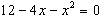(b)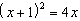(c)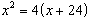14. Solve the following simultaneous equations:

(a)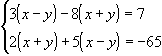(b)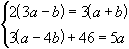15. A bag contains 5 blue, 3 red and 2 green marbles. One marble is drawn at random from the bag. What is the probability that it is

(a) blue (b) red (c) not green (d) blue or green (e) not red nor blue ?